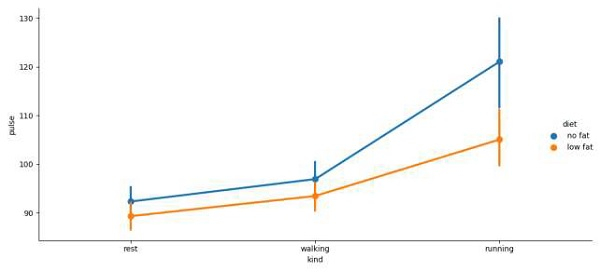# How to change the figuresize using Seaborn factorplot in Matplotlib?

To change the figuresize using Seaborn factorplot, we can take the following Steps −

• Using factorplot() method, change figure size by customising the size and aspect values.

• To display the figure, use the show() method.

## Example

import seaborn as sns
from matplotlib import pyplot as plt
plt.rcParams["figure.figsize"] = [7.50, 3.50]
plt.rcParams["figure.autolayout"] = True
plt.show()